Courses

# IIFT Data Interpretation MCQ - 2

## 18 Questions MCQ Test IIFT Mock Test Series 2020 | IIFT Data Interpretation MCQ - 2

Description
This mock test of IIFT Data Interpretation MCQ - 2 for CAT helps you for every CAT entrance exam. This contains 18 Multiple Choice Questions for CAT IIFT Data Interpretation MCQ - 2 (mcq) to study with solutions a complete question bank. The solved questions answers in this IIFT Data Interpretation MCQ - 2 quiz give you a good mix of easy questions and tough questions. CAT students definitely take this IIFT Data Interpretation MCQ - 2 exercise for a better result in the exam. You can find other IIFT Data Interpretation MCQ - 2 extra questions, long questions & short questions for CAT on EduRev as well by searching above.
QUESTION: 1

### Group Question Answer the following question based on the information given below. Analyze the charts given below that show the energy consumption and energy production of XYZ country from various sources. The difference in production and consumption of energy from any source is bridged through external trade (i.e. export or import) of energy from the same source. All the values are given in Terawatt-hours (Twh).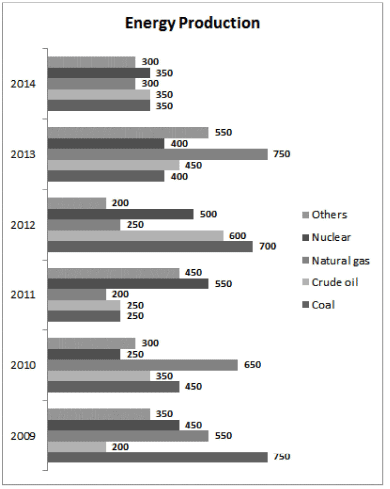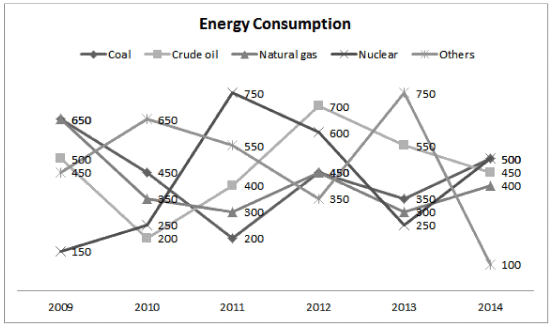Q. Which year registered the highest external trade in the energy sector?

Solution:

External trade = | Imports coal | + | Imports crude oil I + I Imports Natural gas I + I Imports Nuclear I + I Imports others I
Imports = Consumption - Production
In the equation above, exports have also been considered as imports i.e. a negative value of imports would actually mean an export.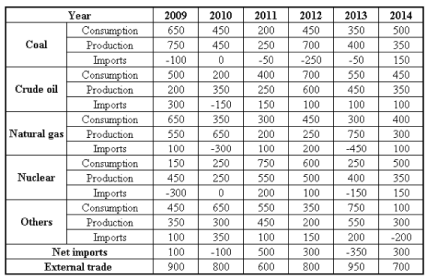From the table, the external trade is highest in the year 2013.

QUESTION: 2

### Analyze the charts given below that show the energy consumption and energy production of XYZ country from various sources. The difference in production and consumption of energy from any source is bridged through external trade (i.e. export or import) of energy from the same source. All the values are given in Terawatt-hours (Twh).Q. In which year are the net imports of all the categories taken together the lowest?

Solution:

Consider the solution to the first question.
Among the given options, net imports are lowest in 2009.
Hence, option 1.

QUESTION: 3

### Analyze the charts given below that show the energy consumption and energy production of XYZ country from various sources. The difference in production and consumption of energy from any source is bridged through external trade (i.e. export or import) of energy from the same source. All the values are given in Terawatt-hours (Twh).Q. Which of the following statement(s) is/are true? I. In each year, net exports are always less than the external trade. II. Coal is always exported. III. Net import of energy from at least one source is nil from 2009-2014.

Solution:

Net exports = - net imports
From the table, it is always less than the external trade.
Thus, statement I is true.
Import of coal in 2014 is 150TWh.
Thus, statement II is false.
Net import of nuclear energy = -300 + 0 + 200 + 100 -150 + 150 = 0
Thus, statement III is true.

QUESTION: 4

Group Question
Answer the following question based on the information given below.

Eight friends Raju, Ravi, Ramesh, Rahul, Arjun, Arvind, Anish and Atul are skilled at making Handicraft items. In order to make money using their skills, they decide to make a certain number of handicraft items and sell them at a Handicraft Expo. They decided to minimize time and maximize their productivity by forming two groups. The people whose names start with the letter ‘R’ form Group 1 and the people whose names start with the letter ‘A’ form Group 2. Each person from Group 1 forms a team each person from Group 2 to produce a certain number of handicraft items of a particular type, the details of which are given in the tables below.
Table 1 gives details of the type of handicraft item produced by each team.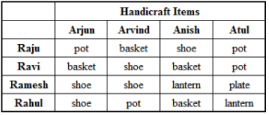Table 2 gives details of the number of handicraft items produced by each team.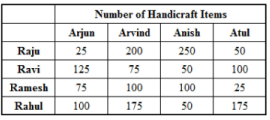Table 3 gives the cost price and selling price of one item of each type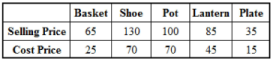The money (Cost Price and Profit) is shared according to the number of items each one has worked on. For example, if Rahul and Arvind made 175 pots, the total cost price and the profit made by selling those pots would be shared equally.

Q. Who has worked on the maximum number of handicraft items?

Solution:

The number of handicraft items worked on by any person is directly given in table 2.
Raju, Rahul, Arvind and Anish have worked on 525, 500, 550 and 5
450 items respectively.
Thus, among these four people, Arvind has worked on the maximum number of handicraft items.
Hence, option 3.

QUESTION: 5

Eight friends Raju, Ravi, Ramesh, Rahul, Arjun, Arvind, Anish and Atul are skilled at making Handicraft items. In order to make money using their skills, they decide to make a certain number of handicraft items and sell them at a Handicraft Expo. They decided to minimize time and maximize their productivity by forming two groups. The people whose names start with the letter ‘R’ form Group 1 and the people whose names start with the letter ‘A’ form Group 2. Each person from Group 1 forms a team each person from Group 2 to produce a certain number of handicraft items of a particular type, the details of which are given in the tables below.
Table 1 gives details of the type of handicraft item produced by each team.Table 2 gives details of the number of handicraft items produced by each team.Table 3 gives the cost price and selling price of one item of each typeThe money (Cost Price and Profit) is shared according to the number of items each one has worked on. For example, if Rahul and Arvind made 175 pots, the total cost price and the profit made by selling those pots would be shared equally.

Q. For how many teams was the total selling price more than Rs. 15,000?

Solution:

The total selling prices for the teams are given in the table below: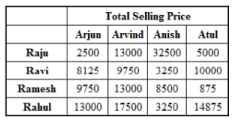The total selling price was more than Rs. 15,000 for 2 teams: Raju-Anish and Rahul-Arvind.

QUESTION: 6

Eight friends Raju, Ravi, Ramesh, Rahul, Arjun, Arvind, Anish and Atul are skilled at making Handicraft items. In order to make money using their skills, they decide to make a certain number of handicraft items and sell them at a Handicraft Expo. They decided to minimize time and maximize their productivity by forming two groups. The people whose names start with the letter ‘R’ form Group 1 and the people whose names start with the letter ‘A’ form Group 2. Each person from Group 1 forms a team each person from Group 2 to produce a certain number of handicraft items of a particular type, the details of which are given in the tables below.
Table 1 gives details of the type of handicraft item produced by each team.Table 2 gives details of the number of handicraft items produced by each team.Table 3 gives the cost price and selling price of one item of each typeThe money (Cost Price and Profit) is shared according to the number of items each one has worked on. For example, if Rahul and Arvind made 175 pots, the total cost price and the profit made by selling those pots would be shared equally.

Q. For how many teams was the ratio of the total cost price to the total selling price less than 0.5?

Solution:

The ratio of cost price to selling price is less than 0.5 for only baskets and plates.
Hence, any team that has made baskets or plates satisfies the given conditions. 4 teams have made baskets and 1 team has made plates i.e. 5 teams satisfy the condition.
Hence, option 3.

QUESTION: 7

Eight friends Raju, Ravi, Ramesh, Rahul, Arjun, Arvind, Anish and Atul are skilled at making Handicraft items. In order to make money using their skills, they decide to make a certain number of handicraft items and sell them at a Handicraft Expo. They decided to minimize time and maximize their productivity by forming two groups. The people whose names start with the letter ‘R’ form Group 1 and the people whose names start with the letter ‘A’ form Group 2. Each person from Group 1 forms a team each person from Group 2 to produce a certain number of handicraft items of a particular type, the details of which are given in the tables below.
Table 1 gives details of the type of handicraft item produced by each team.Table 2 gives details of the number of handicraft items produced by each team.Table 3 gives the cost price and selling price of one item of each typeThe money (Cost Price and Profit) is shared according to the number of items each one has worked on. For example, if Rahul and Arvind made 175 pots, the total cost price and the profit made by selling those pots would be shared equally.

Q. Which of the following statement(s) is/are true?
I. The total profit earned by Atul is Rs. 6,000
II. The total selling price of all the items that Ramesh has worked on is Rs. 32,125
III. The team of Raju and Arjun had the lowest total selling price of the items they had produced.

Solution:

Statement III can be directly verified from the solutions to one of the earlier questions.
The team of Ramesh-Atul has the lowest selling price.
Hence, statement III is false.
Hence, options 2 and 4 can be eliminated.
Since II is present in both the remaining options, you can directly assume it to be true.
Hence, just verify I.
The total profit earned by Atul = (50/2) * 30 + (100/2) x 30 + (25/2 x 20) + (175/2 x 40) = Rs. 6,000
Thus, statement I is true.
Hence, option 1.

QUESTION: 8

Eight friends Raju, Ravi, Ramesh, Rahul, Arjun, Arvind, Anish and Atul are skilled at making Handicraft items. In order to make money using their skills, they decide to make a certain number of handicraft items and sell them at a Handicraft Expo. They decided to minimize time and maximize their productivity by forming two groups. The people whose names start with the letter ‘R’ form Group 1 and the people whose names start with the letter ‘A’ form Group 2. Each person from Group 1 forms a team each person from Group 2 to produce a certain number of handicraft items of a particular type, the details of which are given in the tables below.
Table 1 gives details of the type of handicraft item produced by each team.Table 2 gives details of the number of handicraft items produced by each team.Table 3 gives the cost price and selling price of one item of each typeThe money (Cost Price and Profit) is shared according to the number of items each one has worked on. For example, if Rahul and Arvind made 175 pots, the total cost price and the profit made by selling those pots would be shared equally.

Q. For which type of handicraft was the total number of items 1Marks produced the highest?

Solution:

The total number of items for each type of handicraft is:
Baskets = 125 + 200 + 50 + 50 = 425
Shoes = 75 + 100 + 75 + 100 + 250 = 600
Pots = 25 + 175 + 50 + 100 = 350
Lanterns = 100 + 175 = 275
Hence, option 2.

QUESTION: 9

Group Question
Answer the following question based on the information given below.

Year-wise break-up of number of educational institutions in India: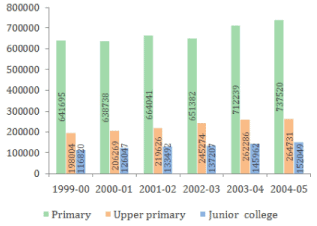Q. Which of the following year has seen the maximum percentage growth of Upper primary schools over the previous year?

Solution:

Percentage growth of Upper primary schools: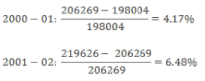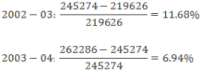The year 2002-03 has seen the maximum growth.
Hence, option 3.

QUESTION: 10

Year-wise break-up of number of educational institutions in India:Q. Which of the following statements is definitely true?

Solution:

Option (1):

Percentage share of Upper primary institutions in 1999 - 00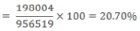Percentage share of Upper primary institutions in 2000 - 01 =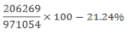Increase = 21.24 - 20.70 = 0.54%
Percentage share of Prim ary institutions in 1999 - 00 =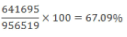Percentage share of Prim ary institutions in 2000 - 01 =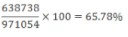Decrease = 67.09 - 65.78 = 1.31%

Since the increase in percentage share of Upper primary institutions in 2000-01 is less than the decrease in percentage share of Primary institutions in the same period, the statement is false.

Option (2):

Percentage share o f Upper prim ary institutions in 2003 - 04 =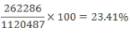Percentage share o f Upper prim ary institutions in 2004 - 05 =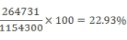Decrease = 23.41 - 22.93 = 0.48%
Percentage share o f Prim ary institutions in 2003 - 04 =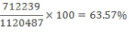Percentage share o f Prim ary institutions in 2004 - 05 =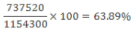Increase = 63.89 - 63.57 = 0.32%

Since the decrease in percentage share of Upper primary institutions in 2004-05 is more than the increase in percentage share of Primary institutions in the same period, the statement is false.

Option (3):

From option (1), we have already seen that the year 2000-01 has also seen a decline in the percentage share of primary institutions, so the statement is false.

Option (4):

Percentage sh are o f Junior colleges in 2002 -03 =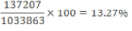Percentage share of Junior colleges in 2003 - 04 =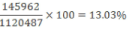Decrease = 13.27 - 13.03 = 0.24%

Percentage share of Primary institutions in 2002 - 03 =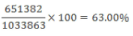Percentage share of Primary institutions in 2003 - 04 =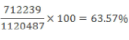Increase = 63.57 - 63.00 = 0.57%

Since the decrease in percentage share of Junior colleges in total in the year 2003-04 over the previous years is less than the increase in percentage share of primary institutions in the same period, the statement is true.

Hence, option 4.

QUESTION: 11

Year-wise break-up of number of educational institutions in India:Q. Which of the following statement(s) is/are definitely true?

Solution:

Option (1):

The statement is false as the number of Primary institutions first
decreased in 2000-01, then increased in 2001-02 and again decreased in 2002-03.
Options (2) and (3) are true.
Hence, option 4.

QUESTION: 12

Year-wise break-up of number of educational institutions in India:Q. What is the number of years in which the total number of Upper primary schools and Junior colleges is less than half of the number of Primary schools?

Solution:

The total number of Upper primary schools and Junior colleges is less than half the number of Primary schools only in the year 1999- 00.
Hence, option 1.

QUESTION: 13

Year-wise break-up of number of educational institutions in India:Q. If in the year 2005-06, the number of Upper primary schools grows by the highest percentage growth recorded in the last 6 years (for Upper primary schools), then what is the expected number of Upper primary schools in 2005-06?

Solution:

In the solution to the 1st problem of this set, it is has been found that the highest percentage growth for Upper primary schools was 11.68% in 2002-03.
Hence, the number of Upper primary schools in 2005-06 = 264731 x 1.1168 = 295651.58*295652
Hence, option 2.

QUESTION: 14

Group Question
Answer the following question based on the information given below.

The table below shows production area, production quantity, export quantity, import quantity, export and import value of dry fruits over the period of five years in a country.
The production is consumed in two ways - export and domestic consumption.
The export and import values in crores are in the local currency of the country.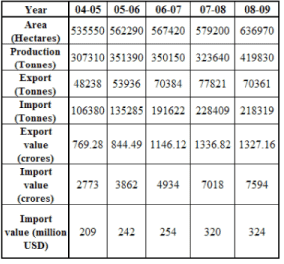Q. Approximately, what percentage of domestic consumption are imports of dry fruits during 2005-2006?

Solution:

In 2005-2006, the domestic consumption is 351390 - 53936 = 297454 tonnes

Imports in that year = 135285
The imports as a percentage of domestic consumption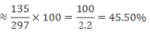Hence, option 2.

QUESTION: 15

The table below shows production area, production quantity, export quantity, import quantity, export and import value of dry fruits over the period of five years in a country.
The production is consumed in two ways - export and domestic consumption.
The export and import values in crores are in the local currency of the country.Q. What is the value of exports in 2008-09 in millions of US dollars, considering the same foreign exchange value as imports in that year?

Solution:

As 7594 crores in local currency correspond to 324 million USD, Each unit of local currency in 2008-2009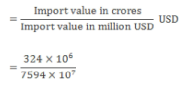The value of exports in the year 2008-2009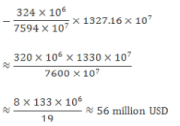The closest answer is option 2.
Hence, option 2.

QUESTION: 16

The table below shows production area, production quantity, export quantity, import quantity, export and import value of dry fruits over the period of five years in a country.
The production is consumed in two ways - export and domestic consumption.
The export and import values in crores are in the local currency of the country.Q. Which of the following statements is true?

Solution:

Consider option 1:
Production per hectare in 2004-2005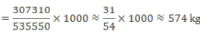The statement in option 1 is false.

Consider option 2:

Production per hectare in 2008-2009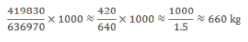The statement in option 2 is false.

Consider option 3: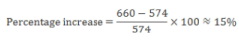The statement in option 3 is true.

Hence, option 3.

QUESTION: 17

The table below shows production area, production quantity, export quantity, import quantity, export and import value of dry fruits over the period of five years in a country.
The production is consumed in two ways - export and domestic consumption.
The export and import values in crores are in the local currency of the country.Q. Which of the following statements is true?

Solution: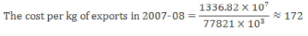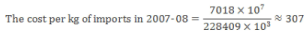The difference in costs of exports and imports = 307 - 172 = Rs. 135

Hence, option 1.

QUESTION: 18

The table below shows production area, production quantity, export quantity, import quantity, export and import value of dry fruits over the period of five years in a country.
The production is consumed in two ways - export and domestic consumption.
The export and import values in crores are in the local currency of the country.Q. Which of the following statements is false?

Solution:

Consider option 1:
Total number of hectares required in 2013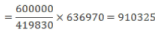Additional area needed = 910325 - 636970 = 273355 hectares or 2.73 lakh hectares.

Consider option 2:

Total number of hectares required in 2013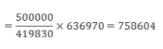Additional area needed = 758604 - 636970 = 121634 hectares or 1.22 lakh hectares.

Consider option 3:

Total number of hectares required in 2013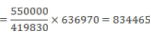Additional area needed = 834465 - 636970 = 197495 hectares or 1.98 lakh hectares.

Hence, option 3.